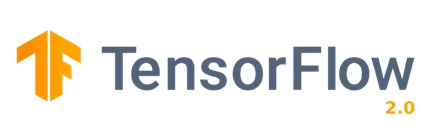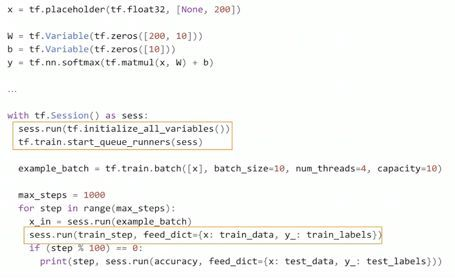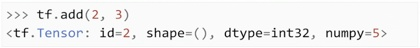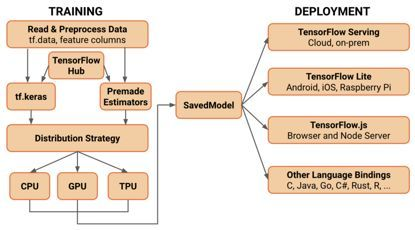## 关于TensorFlow 2.0，这里有你想知道的一切 原TensorFlow是谷歌2015年开源的通用高性能计算库。最初主要是为构建神经网络(NNs)提供高性能的API。然而，随着时间的推移和机器学习(ML)社区的兴起，TensorFlow已经发展为一个完整的机器学习生态系统。TensorFlow自诞生以来变化巨大。TensorFlow 2.0目前处于测试阶段，与TF1.x相比有了许多变化。下面是主要的几个变化。

#### 1. 默认状态的Eager ExecutionTF 1.x代码示例Tensorflow 2.0代码示例

TensorFlow 2.0代码看起来很像NumPy代码。事实上，TensorFlow和NumPy的对象可以很容易地切换。因此不必担心占位符、会话、feed_dictionaties等问题。

#### 2. API清理

tf.slim，tf.layers，tf.contrib.layers，tf.keras都是可以用来构建神经网络的API。不包括TF1.x中的序列到API序列。大多数情况下，每种情况下要选择哪一种尚不明确。

#### 3. 初学者API

```model= tf.keras.models.Sequential()
model.add(Flatten(input_shape=
(IMAGE_HEIGHT,IMAGE_WIDTH)))
model.add(Dense(units=32,activation='relu'))
model.add(Dropout(0.3))
model.add(Dense(units=32,activation='relu'))
model.add(Dense(units=10,activation='softmax'))
#Configures the model for training.
#Define the model optimizer, the loss
function and the accuracy metrics
model.compile(optimizer='adam',
loss='sparse_categorical_crossentropy',
metrics=['accuracy'])
model.summary()```

#### 4. Keras-Tuner

Keras-tuner是一个对Keras模型进行超参数调整的专用库。在撰写本文时，该库处于α之前的状态，但是使用tf.keras和TensorFlow 2.0β可以在Colab上很好地运行。

```defbuild_model(hp):
# define the hyper parameter ranges for
thelearning rate, dropout and hidden unit
hp_units = hp.Range('units', min_value=32,max_value=128, step=32)
hp_lr = hp.Choice('learning_rate',values=[1e-2, 1e-3, 1e-4])
hp_dropout = hp.Choice('dropout',values=[0.1,0.2,0.3])

# build a Sequential model
model = keras.Sequential()
model.add(Flatten(input_shape=(IMAGE_HEIGHT,IMAGE_WIDTH)))
model.add(Dense(units=hp_units,activation='relu'))
model.add(Dropout(hp_dropout))
model.add(Dense(units=32, activation='relu'))
model.add(layers.Dense(10,activation='softmax'))
# compile and return the model model.compile(optimizer=keras.optimize
rs.Adam(hp_lr),
loss='sparse_categorical_crossentropy',
metrics=['accuracy'])

return model
#create a Random Search tunertuner= RandomSearch(
build_model,
objective='val_accuracy', # define themetric to be optimized over
max_trials=3,
executions_per_trial=1,
directory='my_logs')
# define the outputlog/checkpoints folder
# start hyper-parameter optmization searchtuner.search(x_train,y_train,
epochs=2,
validation_data=(x_test, y_test))```

#### 5. 高级API

```class Model(tf.keras.Model):
def__init__(self):
# Define thelayers here
super(Model,self).__init__()
self.conv1 =Conv2D(filters=8, kernel_size=4, padding="same",
strides=1,input_shape=(IMAGE_HEIGHT,IMAGE_WIDTH,IMAGE_DEPTH))
self.conv2 =Conv2D(filters=16, kernel_size=4, padding="same", strides=1)
self.pool =MaxPool2D(pool_size=2, strides=2, padding="same")
self.flat =Flatten()
elf.probs =Dense(units=N_CLASSES, activation='softmax', name="output")
def call(self,x):

# Define theforward pass
net =self.conv1(x)
net =self.pool(net)
net =self.conv2(net)
net =self.pool(net)
net =self.flat(net)
net =self.probs(net)
return net

defcompute_output_shape(self, input_shape):
# You need tooverride this function if you want to use the subclassed model
# as part of afunctional-style model.
# Otherwise,this method is optional.
shape =tf.TensorShape(input_shape).as_list()
shape[-1] =self.num_classes
returntf.TensorShape(shape)```

· 正推法

· 损失函数求值

· 逆推法

· 梯度下降法

#### 7. 设置TensorBoard

fit()可以用于序列化API和子类化API。

```# Load theTensorBoard notebook extension%load_exttensorboard
# create thetensorboard callbacktensorboard= TensorBoard
(log_dir='logs/{}'.format(time.time()), histogram_freq=1)
# train themodelmodel.fit(x=x_train,

y=y_train,
epochs=2,
validation_data=(x_test, y_test),
callbacks=[tensorboard])
# launchTensorBoard%tensorboard--logdir logs```

· EarlyStopping:顾名思义，它建立了一个规则——当监控量停止增加时，就停止培训。

· ReduceLROnPlateau:当测度停止增加时，降低学习速率。

· TerminateOnNaN:遇到NaN丢失时终止培训。

· LambdaCallback:用于创建简单的自定义联机回调。

#### 8. EagerCode的提取性能

```@tf.functiondeftrain_step(images, labels):
with tf.GradientTape() as tape:
# forward pass
predictions = model(images)

# compute the loss
loss = cross_entropy(tf.one_hot(labels,N_CLASSES), predictions)
# get the gradients w.r.t the model's weights
gradients = tape.gradient(loss,model.trainable_variables)

# perform a gradient descent step
optimizer.apply_gradients(zip(gradients,model.trainable_variables))
# accumulates the training loss and accuracy  train_loss(loss)
train_accuracy(labels, predictions)```

#### 9. 保存和修复模型

TF1.x对于如何保存/加载经过培训的模型以用于生产缺乏一致的标准。TF2.0试图通过定义单个API来解决这个问题。

TF2.0保存模型的方法不多，而是对一个称为SavedModel的抽象进行标准化。

```# serialize your model to a SavedModel object
# It includes the entire graph, all variables
andweightsmodel.save('/tmp/model', save_format='tf')
# load your saved

modelmodel = tf.keras.
models.load_
model('/tmp/model')```

SavedModel与Tensorflow生态系统结合到一起。换句话说，能够将其分配到许多不同的设备，包括移动电话、边缘设备和服务器。#### 10. 转换至TF-Lite

```# create a TF Lite converter
converter
=tf.lite.TFLiteConverter.from_keras_mode
l(model)

# performs model quantization to reduce
the size of themodel and improve latency

converter.optimizations
=[tf.lite.Optimize.OPTIMIZE_FOR_SIZE]
tflite_model = converter.convert()```

#### 11. 转换至TensorFlow.js

```!tensorflowjs_converter\
--input_format=tf_saved_model \
--saved_model_tags=serve \
--output_format=tfjs_graph_model \
/tmp/model \
/tmp/web_model```

#### 结语© 著作权归作者所有

TensorFlow 2.0深度强化学习指南

【方向】
01/24
0
0
TensorFlow 2.0开发者测试版来啦，正式版推出指日可待

01/11
0
0

Alice熹爱学习
01/14
0
0
TensorFlow从1到2（一）续讲从锅炉工到AI专家

04/07
0
0

2018/10/12
0
0

64.监控平台介绍 安装zabbix 忘记admin密码

19.1 Linux监控平台介绍 19.2 zabbix监控介绍 19.3/19.4/19.6 安装zabbix 19.5 忘记Admin密码如何做 19.1 Linux监控平台介绍： 常见开源监控软件 ~1.cacti、nagios、zabbix、smokeping、ope...

oschina130111

13
0

7
0
DNS-over-HTTPS 的下一代是 DNS ON BLOCKCHAIN

12
0
CC攻击带来的危害我们该如何防御？

12
0

8
0